# Volume + work - math problems

#### Number of problems found: 30

• The dam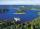The water reservoir is filled with first tributary for 25 hours second tributary for 35 hours. Some time were both inlets open, then a second tributary closed and the tank was filled in four hours. What time water flowed from both tributaries? (Expressed
• Tank 9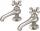The tank with volume V is filled with one pump for three hours and by the second pump for 5 hours. When both pumps run simultaneously, calculate: a) how much of the total volume of the tank is filled in one hour b) for how long is the tank full
• Tank No 8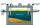Tank is filled by one inlet valve with a flow rate of 12 liters per second in 72 minutes. How long take the tank to fill, if we open half an hour after one more inlet?
• Water pool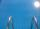Pool with volume 990hl completely filled, if water flows by one tap 8 hours and by second tap 6 hours. First tap give 10hl more than second per hour. How many hl flows in each of them in an hour?
• Pumps 3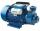Two pumps of the same power fill the garden pool for 10 hours. How many of these pumps would have to use if we want to shorten the filling of the pool to four hours?
• 4 pipes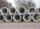The tank flows out by 4 pipes in 6 hours 120 hl water. How much water flows out of 5 pipes of the same diameter in 14 hours?
• Pumps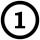First pump flows 16 liters per second into the basin by the second pump 75% of the first and by third pump half more than the second. How long will take fill basin by all three pumps simultaneously volume 15 m3 (cubic meters)?
• Pool 2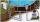The first supply by the pool fill for five hours and the second fill for six hours, drain should be drained for 15 hours. For how many hours the pool is full, when we open both inlet now and outlet open two hours later?
• PoolIf water flows into the pool by two inlets, fill the whole for 19 hours. The first inlet filled pool 5 hour longer than the second. How long pool take to fill with two inlets separately?
• PipesThe water pipe has a cross-section 1087 cm2. An hour has passed 960 m3 of water. How much water flows through the pipe with cross-section 300 cm2 per 9 hours if water flows the same speed?

We apologize, but in this category are not a lot of examples.
Do you have an exciting math question or word problem that you can't solve? Ask a question or post a math problem, and we can try to solve it.

We will send a solution to your e-mail address. Solved examples are also published here. Please enter the e-mail correctly and check whether you don't have a full mailbox.

Tip: Our volume units converter will help you with the conversion of volume units. Volume - math problems. Work word problems.